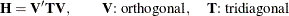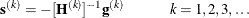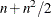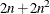### Computational Resources

Since nonlinear optimization is an iterative process that depends on many factors, it is difficult to estimate how much computer time is necessary to find an optimal solution satisfying one of the termination criteria. You can use the MAXTIME=, MAXITER=, and MAXFUNC= options to restrict the amount of CPU time, the number of iterations, and the number of function calls in a single run of PROC NLMIXED.

In each iteration k, the NRRIDG technique uses a symmetric Householder transformation to decompose theHessian matrix,to compute the (Newton) search direction,The TRUREG and NEWRAP techniques use the Cholesky decomposition to solve the same linear system while computing the search direction. The QUANEW, DBLDOG, CONGRA, and NMSIMP techniques do not need to invert or decompose a Hessian matrix; thus, they require less computational resources than the other techniques.

The larger the problem, the more time is needed to compute function values and derivatives. Therefore, you might want to compare optimization techniques by counting and comparing the respective numbers of function, gradient, and Hessian evaluations.

Finite-difference approximations of the derivatives are expensive because they require additional function or gradient calls:

• forward-difference formulas

• For first-order derivatives, n additional function calls are required.

• For second-order derivatives based on function calls only, for a dense Hessian,additional function calls are required.

• For second-order derivatives based on function calls only, for a dense Hessian,additional function calls are required.